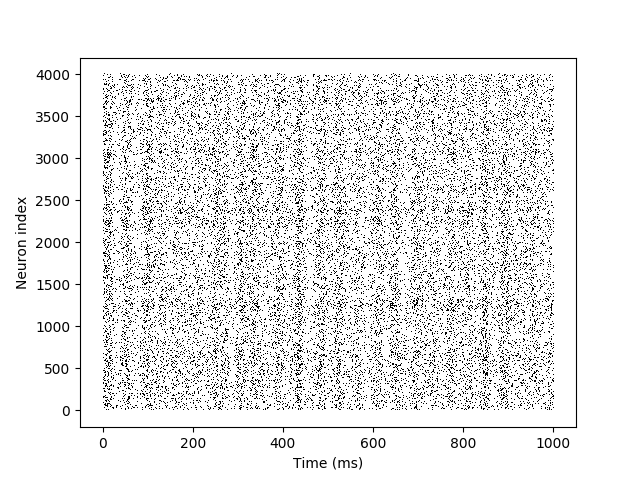# Example: CUBA¶

Note

You can launch an interactive, editable version of this example without installing any local files using the Binder service (although note that at some times this may be slow or fail to open):This is a Brian script implementing a benchmark described in the following review paper:

Simulation of networks of spiking neurons: A review of tools and strategies (2007). Brette, Rudolph, Carnevale, Hines, Beeman, Bower, Diesmann, Goodman, Harris, Zirpe, Natschlager, Pecevski, Ermentrout, Djurfeldt, Lansner, Rochel, Vibert, Alvarez, Muller, Davison, El Boustani and Destexhe. Journal of Computational Neuroscience 23(3):349-98

Benchmark 2: random network of integrate-and-fire neurons with exponential synaptic currents.

Clock-driven implementation with exact subthreshold integration (but spike times are aligned to the grid).

from brian2 import *

taum = 20*ms
taue = 5*ms
taui = 10*ms
Vt = -50*mV
Vr = -60*mV
El = -49*mV

eqs = '''
dv/dt  = (ge+gi-(v-El))/taum : volt (unless refractory)
dge/dt = -ge/taue : volt
dgi/dt = -gi/taui : volt
'''

P = NeuronGroup(4000, eqs, threshold='v>Vt', reset='v = Vr', refractory=5*ms,
method='exact')
P.v = 'Vr + rand() * (Vt - Vr)'
P.ge = 0*mV
P.gi = 0*mV

we = (60*0.27/10)*mV # excitatory synaptic weight (voltage)
wi = (-20*4.5/10)*mV # inhibitory synaptic weight
Ce = Synapses(P, P, on_pre='ge += we')
Ci = Synapses(P, P, on_pre='gi += wi')
Ce.connect('i<3200', p=0.02)
Ci.connect('i>=3200', p=0.02)

s_mon = SpikeMonitor(P)

run(1 * second)

plot(s_mon.t/ms, s_mon.i, ',k')
xlabel('Time (ms)')
ylabel('Neuron index')
show()